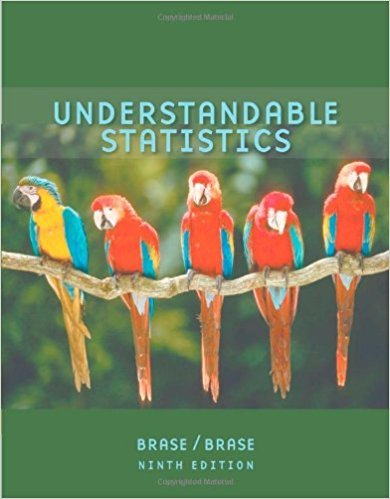×
×

# Solutions for Chapter 6.1: NORMAL DISTRIBUTIONS## Full solutions for Understandable Statistics | 9th Edition

ISBN: 9780618949922Solutions for Chapter 6.1: NORMAL DISTRIBUTIONS

Solutions for Chapter 6.1
4 5 0 368 Reviews
14
4
##### ISBN: 9780618949922

Understandable Statistics was written by and is associated to the ISBN: 9780618949922. Chapter 6.1: NORMAL DISTRIBUTIONS includes 15 full step-by-step solutions. This expansive textbook survival guide covers the following chapters and their solutions. This textbook survival guide was created for the textbook: Understandable Statistics, edition: 9. Since 15 problems in chapter 6.1: NORMAL DISTRIBUTIONS have been answered, more than 34087 students have viewed full step-by-step solutions from this chapter.

Key Statistics Terms and definitions covered in this textbook
• Average run length, or ARL

The average number of samples taken in a process monitoring or inspection scheme until the scheme signals that the process is operating at a level different from the level in which it began.

• Bayes’ estimator

An estimator for a parameter obtained from a Bayesian method that uses a prior distribution for the parameter along with the conditional distribution of the data given the parameter to obtain the posterior distribution of the parameter. The estimator is obtained from the posterior distribution.

• Categorical data

Data consisting of counts or observations that can be classiied into categories. The categories may be descriptive.

• Causal variable

When y fx = ( ) and y is considered to be caused by x, x is sometimes called a causal variable

• Central composite design (CCD)

A second-order response surface design in k variables consisting of a two-level factorial, 2k axial runs, and one or more center points. The two-level factorial portion of a CCD can be a fractional factorial design when k is large. The CCD is the most widely used design for itting a second-order model.

• Central tendency

The tendency of data to cluster around some value. Central tendency is usually expressed by a measure of location such as the mean, median, or mode.

• Comparative experiment

An experiment in which the treatments (experimental conditions) that are to be studied are included in the experiment. The data from the experiment are used to evaluate the treatments.

• Components of variance

The individual components of the total variance that are attributable to speciic sources. This usually refers to the individual variance components arising from a random or mixed model analysis of variance.

• Contingency table.

A tabular arrangement expressing the assignment of members of a data set according to two or more categories or classiication criteria

• Continuous uniform random variable

A continuous random variable with range of a inite interval and a constant probability density function.

• Correlation

In the most general usage, a measure of the interdependence among data. The concept may include more than two variables. The term is most commonly used in a narrow sense to express the relationship between quantitative variables or ranks.

• Correlation matrix

A square matrix that contains the correlations among a set of random variables, say, XX X 1 2 k , ,…, . The main diagonal elements of the matrix are unity and the off-diagonal elements rij are the correlations between Xi and Xj .

• Critical region

In hypothesis testing, this is the portion of the sample space of a test statistic that will lead to rejection of the null hypothesis.

• Cumulative normal distribution function

The cumulative distribution of the standard normal distribution, often denoted as ?( ) x and tabulated in Appendix Table II.

• Decision interval

A parameter in a tabular CUSUM algorithm that is determined from a trade-off between false alarms and the detection of assignable causes.

• Defect concentration diagram

A quality tool that graphically shows the location of defects on a part or in a process.

• Degrees of freedom.

The number of independent comparisons that can be made among the elements of a sample. The term is analogous to the number of degrees of freedom for an object in a dynamic system, which is the number of independent coordinates required to determine the motion of the object.

• Density function

Another name for a probability density function

• Discrete distribution

A probability distribution for a discrete random variable

• Exponential random variable

A series of tests in which changes are made to the system under study

×